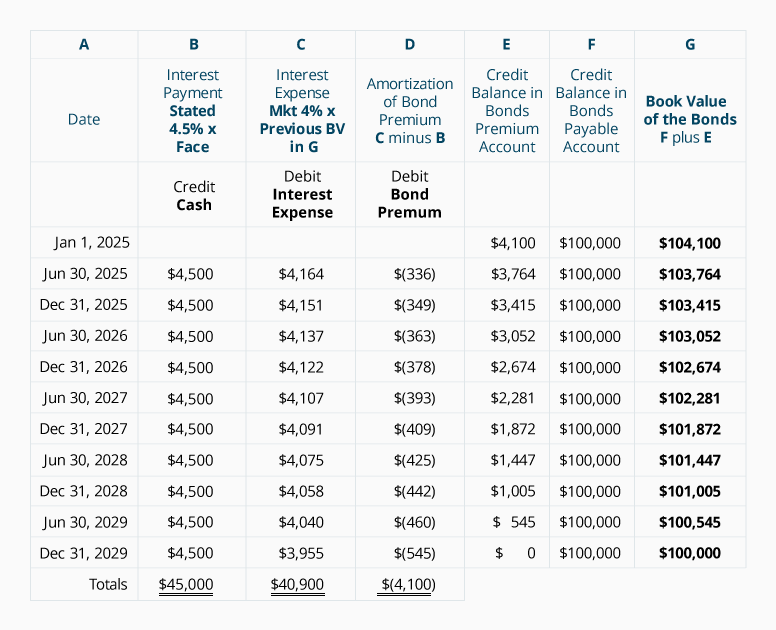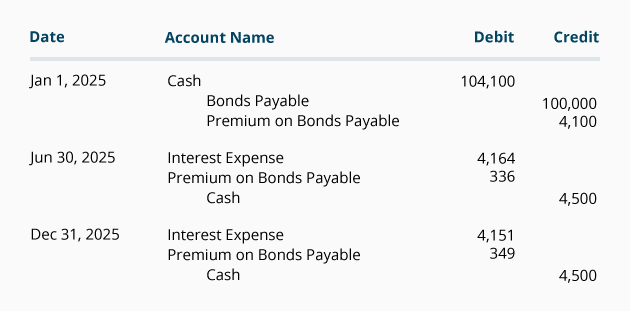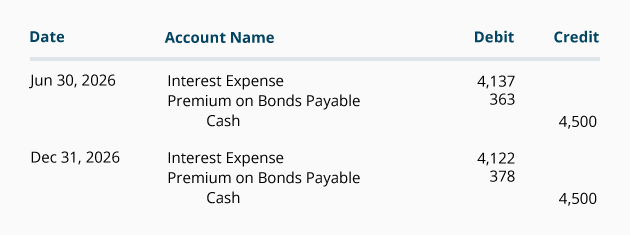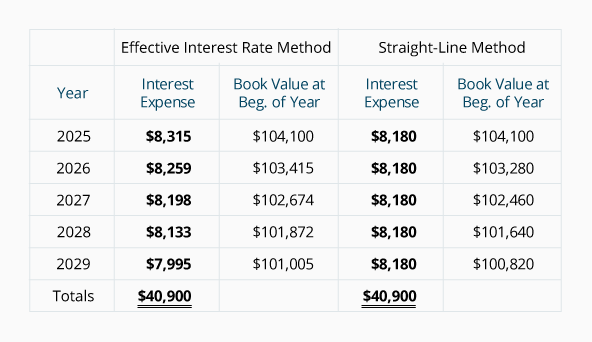# Amortizing Bond Premium with the Effective Interest Rate Method

When a bond is sold at a premium, the amount of the bond premium must be amortized to interest expense over the life of the bond. In other words, the credit balance in the account Premium on Bonds Payable must be moved to the account Interest Expense thereby reducing interest expense in each of the accounting periods that the bond is outstanding.

The preferred method for amortizing the bond premium is the effective interest rate method or the effective interest method. Under the effective interest rate method the amount of interest expense in a given year will correlate with the amount of the bond's book value. This means that when a bond's book value decreases, the amount of interest expense will decrease. In short, the effective interest rate method is more logical than the straight-line method of amortizing bond premium.

Before we demonstrate the effective interest rate method for amortizing the bond premium pertaining to a 5-year 9% \$100,000 bond issued in an 8% market for \$104,100 on January 1, 2022, let's outline a few concepts:

1. The bond premium of \$4,100 must be amortized to Interest Expense over the life of the bond. This amortization will cause the bond's book value to decrease from \$104,100 on January 1, 2022 to \$100,000 just prior to the bond maturing on December 31, 2026.
2. The corporation must make an interest payment of \$4,500 (\$100,000 x 9% x 6/12) on each June 30 and December 31. This means that the Cash account will be credited for \$4,500 on each interest payment date.
3. The effective interest rate method uses the market interest rate at the time that the bond was issued. In our example, the market interest rate on January 1, 2022 was 4% per semiannual period for 10 semiannual periods.
4. The effective interest rate is multiplied times the bond's book value at the start of the accounting period to arrive at each period's interest expense.
5. The difference between Item 2 and Item 4 is the amount of amortization.

The following table illustrates the effective interest rate method of amortizing the \$4,100 premium on a corporation's bonds payable:Please make note of the following points:

• Column B shows the interest payments required in the bond contract: The bond's stated rate of 9% per year divided by two semiannual periods = 4.5% per semiannual period times the face amount of the bond
• Column C shows the interest expense. This calculation uses the market interest rate at the time the bond was issued: The market rate of 8% per year divided by two semiannual periods = 4% semiannually.
• The interest expense in column C is the product of the 4% market interest rate per semiannual period times the book value of the bond at the start of the semiannual period. Notice how the interest expense is decreasing with the decrease in the book value in column G. This correlation between the interest expense and the bond's book value makes the effective interest rate method the preferred method.
• Because the present value factors that we used were rounded to three decimal places, our calculations are not as precise as the amounts determined by use of computer software, a financial calculator, or factors with more decimal places. As a result, the amounts in year 2026 required a small adjustment.

If the company issues only annual financial statements and its accounting year ends on December 31, the amortization of the bond premium can be recorded at the interest payment dates by using the amounts from the schedule above. In our example there was no accrued interest at the issue date of the bonds and there is no accrued interest at the end of each accounting year because the bonds pay interest on June 30 and December 31. The entries for 2022, including the entry to record the bond issuance, are:The journal entries for the year 2023 are:The journal entries for 2024, 2025, and 2026 will also be taken from the schedule above.

### Comparison of Amortization Methods

Below is a comparison of the amount of interest expense reported under the effective interest rate method and the straight-line method. Note that under the effective interest rate method the interest expense for each year is decreasing as the book value of the bond decreases. Under the straight-line method the interest expense remains at a constant annual amount even though the book value of the bond is decreasing. The accounting profession prefers the effective interest rate method, but allows the straight-line method when the amount of bond premium is not significant.Notice that under both methods of amortization, the book value at the time the bonds were issued (\$104,100) moves toward the bond's maturity value of \$100,000. The reason is that the bond premium of \$4,100 is being amortized to interest expense over the life of the bond.

Also notice that under both methods the corporation's total interest expense over the life of the bond will be \$40,900 (\$45,000 of interest payments minus the \$4,100 of premium received from the purchasers of the bond when it was issued.)